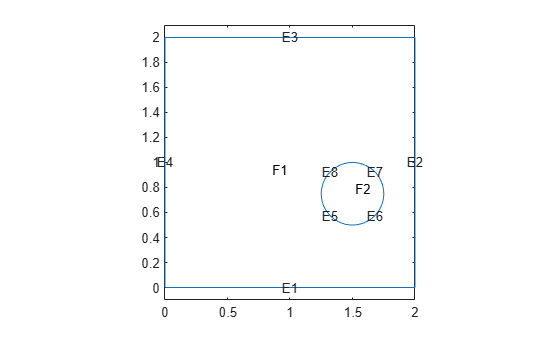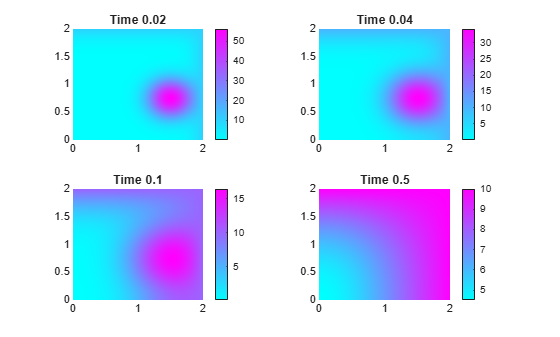TimeDependentResults

Time-dependent PDE solution and derived quantities

Description

A TimeDependentResults object contains the solution of a PDE and its gradients in a form convenient for plotting and postprocessing.

• A TimeDependentResults object contains the solution and its gradient calculated at the nodes of the triangular or tetrahedral mesh, generated by generateMesh.

• Solution values at the nodes appear in the NodalSolution property.

• The solution times appear in the SolutionTimes property.

• The array dimensions of NodalSolution, XGradients, YGradients, and ZGradients enable you to extract solution and gradient values for specified time indices, and for the equation indices in a PDE system.

To interpolate the solution or its gradient to a custom grid (for example, specified by meshgrid), use interpolateSolution or evaluateGradient.

Creation

There are several ways to create a TimeDependentResults object:

• Solve a time-dependent problem using the solvepde function. This function returns a PDE solution as a TimeDependentResults object. This is the recommended approach.

• Solve a time-dependent problem using the parabolic or hyperbolic function. Then use the createPDEResults function to obtain a TimeDependentResults object from a PDE solution returned by parabolic or hyperbolic. Note that parabolic and hyperbolic are legacy functions. They are not recommended for solving PDE problems.

Properties

expand all

Finite element mesh, returned as a FEMesh Properties object.

Solution values at the nodes, returned as a vector or array. For details about the dimensions of NodalSolution, see Dimensions of Solutions, Gradients, and Fluxes.

Data Types: double
Complex Number Support: Yes

Solution times, returned as a real vector. SolutionTimes is the same as the tlist input to solvepde, or the tlist input to the legacy parabolic or hyperbolic solvers.

Data Types: double

x-component of the gradient at the nodes, returned as a vector or array. For details about the dimensions of XGradients, see Dimensions of Solutions, Gradients, and Fluxes.

Data Types: double
Complex Number Support: Yes

y-component of the gradient at the nodes, returned as a vector or array. For details about the dimensions of YGradients, see Dimensions of Solutions, Gradients, and Fluxes.

Data Types: double
Complex Number Support: Yes

z-component of the gradient at the nodes, returned as a vector or array. For details about the dimensions of ZGradients, see Dimensions of Solutions, Gradients, and Fluxes.

Data Types: double

Object Functions

 evaluateCGradient Evaluate flux of PDE solution evaluateGradient Evaluate gradients of PDE solutions at arbitrary points interpolateSolution Interpolate PDE solution to arbitrary points

Examples

collapse all

Solve a parabolic problem with 2-D geometry.

Create and view the geometry: a square with a circular subdomain.

% Square centered at (1,1)
rect1 = [3;4;0;2;2;0;0;0;2;2];
% Circle centered at (1.5,0.5)
circ1 = [1;1.5;.75;0.25];
% Append extra zeros to the circle
circ1 = [circ1;zeros(length(rect1)-length(circ1),1)];
gd = [rect1,circ1];
ns = char('rect1','circ1');
ns = ns';
sf = 'rect1+circ1';
[dl,bt] = decsg(gd,sf,ns);
pdegplot(dl,'EdgeLabels','on','FaceLabels','on')
axis equal
ylim([-0.1,2.1])Include the geometry in a PDE model.

model = createpde();
geometryFromEdges(model,dl);

Set boundary conditions that the upper and left edges are at temperature 10.

applyBoundaryCondition(model,'dirichlet','Edge',[2,3],'u',10);

Set initial conditions that the square region is at temperature 0, and the circle is at temperature 100.

setInitialConditions(model,0);
setInitialConditions(model,100,'Face',2);

Define the model coefficients.

specifyCoefficients(model,'m',0,'d',1,'c',1,'a',0,'f',0);

Solve the problem for times 0 through 1/2 in steps of 0.01.

generateMesh(model,'Hmax',0.05);
tlist = 0:0.01:0.5;
results = solvepde(model,tlist);

Plot the solution for times 0.02, 0.04, 0.1, and 0.5.

sol = results.NodalSolution;
subplot(2,2,1)
pdeplot(model,'XYData',sol(:,3))
title('Time 0.02')
subplot(2,2,2)
pdeplot(model,'XYData',sol(:,5))
title('Time 0.04')
subplot(2,2,3)
pdeplot(model,'XYData',sol(:,11))
title('Time 0.1')
subplot(2,2,4)
pdeplot(model,'XYData',sol(:,51))
title('Time 0.5')Introduced in R2016a

Partial Differential Equation Toolbox Documentation평가판 신청